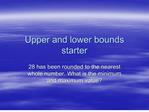DownloadDownload PresentationUpper and lower bounds starter

# Upper and lower bounds starter

Download Presentation## Upper and lower bounds starter

- - - - - - - - - - - - - - - - - - - - - - - - - - - E N D - - - - - - - - - - - - - - - - - - - - - - - - - - -
##### Presentation Transcript

1. Upper and lower bounds starter 28 has been rounded to the nearest whole number. What is the minimum and maximum value?

2. Upper and lower bounds The lower bound is 27.5 and the upper bound is 28.5 To find the upper and lower bounds we added and subtracted 0.5 because this is half of one whole number

3. Upper and lower bounds 240 has been rounded to the nearest ten. What are the minimum and maximum values? What is the quick way of working out the answer?

4. Upper and lower bounds The lower bound is 235 and the upper bound is 245 The quick way to work out the answer is to add and subtract 5 because 5 is half of ten.

5. Upper and lower bound If a number is rounded to the nearest 100 what do we add and subtract to find the minimum and maximum values? If a number has been rounded to the nearest one tenth what do we add and subtract to find the minimum and maximum values? If the number has been rounded to the nearest ten thousand what do we add and subtract this time?

6. Upper and lower bound When rounded to the nearest 100 we add and subtract 50 When rounded to the nearest one tenth we add and subtract 0.05 When rounded to the nearest ten thousand we add and subtract 5,000

7. Write down the upper and lower bounds with the following: 56 to the nearest whole number 7.8 to the nearest tenth 3,400 to the nearest thousand 5.17 to the nearest hundredth 8,000,000 to the nearest million 570,000 to two significant figures 0.00006 to one significant figure 32,800,000,000 to three significant figures

8. Upper and lower bounds Objective: To do calculations involving maximum and minimum using the four arithmetic operations

9. A rectangle is measured as 5 cm by 8cm to the nearest cm. Write down the minimum and maximum values for the lengths. Calculate the minimum and maximum values for the perimeter of the rectangle. Calculate the minimum and maximum values for the area of the rectangle. Minimum and maximum lengths are: 4.5cm and 5.5cm 7.5cm and 8.5cm

10. A rectangle is measured as 5cm by 8cm to the nearest cm The perimeter is found by adding all four sides together: Minimum perimeter is 4.5 + 7.5 + 4.5 + 7.5 which is 24cm. Maximum perimeter is 5.5 + 8.5 + 5.5+ 8.5 which is 28cm.

11. A rectangle is measured as 5cm by 8cm to the nearest cm Minimum area is 4.5 X 7.5 = 33.75 Maximum area is 5.5 X 8.5 = 46.75

12. Addition and multiplication When adding numbers together the minimum value is found by adding the lower bounds together and the maximum value is found by adding the upper bounds. When multiplying the minimum is found by multiplying the lower bounds and the maximum is found by multiplying the upper bounds

13. In a month Fred earns �1200 to the nearest hundred pounds and Mary earns �1500 to nearest hundred What is the minimum and maximum values? What is the minimum and maximum difference in their earnings? Minimum and maximum values are: Fred: �1150 and �1250 Mary: �1450 and �1550

14. Fred earns �1200 and Mary earns �1500 to nearest hundred pounds Minimum difference in earnings will be found as �1450 - �1250 = �200. Maximum difference in earning will be found as �1550 -�1150 = �400.

15. Subtraction To find the minimum difference subtract an upper bound from a lower bound. To find the maximum difference subtract a lower bound from an upper bound.

16. Billy travelled 700 miles to nearest hundred miles in 20 hours rounded to nearest hour. Write down the upper and lower bounds for the mileage and the number of hours. Calculate the minimum and maximum average speed. Minimum and maximum values are: 650 miles and 750 miles 19.5 hrs and 20.5 hrs

17. Billy travelled 700 miles in 20 hours Minimum average speed is found by dividing 650 by 20.5 which is 31.7 mph Maximum average speed is found by dividing 750 by 19.5 which is 38.5 mph

18. Division To calculate the minimum value divide the lower bound by upper bound To calculate the maximum value divide the upper bound by lower bound

19. Advice If you can not work out whether to use upper or lower bounds in a question work out all the possibilities!!!!

20. Plenary Volume of a cuboid 6cm by 4cm by 7cm when lengths rounded to nearest whole number. Three people earn �3,000 �5,000 and �7,000 rounded to nearest thousand. What is the total and average earnings? The distance between Sheerness and Canterbury is 30 miles to nearest mile. What is the distance between the places?